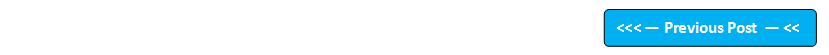## Blog Pages

### 174. 'double' Data Type arraysTill now, we have used integer data type arrays. But now, lets explore the other data types in arrays like double, char, strings etc. To start with, lets implement double data type arrays.

double defined arrays can store more than double type values as shown in the below example.

Example -

double a[] = new double;

a = 1.5 ;
a = 2.0 ;
a = 3.9 ;

The above example is a representation of single dimensional double type arrays. We can also implement 2 dimensional, 3 dimensional arrays using double data type.But in this post, I will only implement single dimensional double data type array.

Lets implement this on Eclipse IDE -

1. Launch Eclipse IDE, create a new Java Project 'Project Arrays' as shown below -

2. Create a Java Class 'DoubleTypeArray' with main( ) method as shown below -

3. Write the below shown program to print the sum of array elements of double type as shown below -

4. Run the java program and observe that the sum of the array elements in displayed in the output as shown below -

This is how we implement the double type arrays.

Lets implement the char type arrays in the next post.